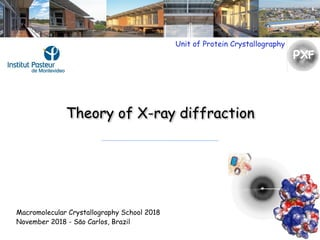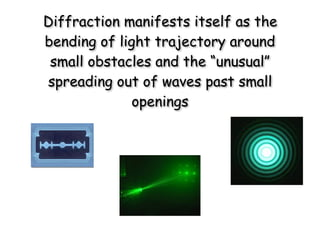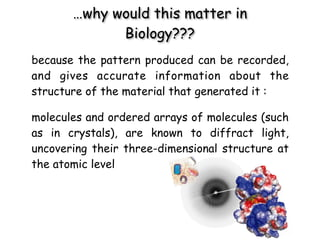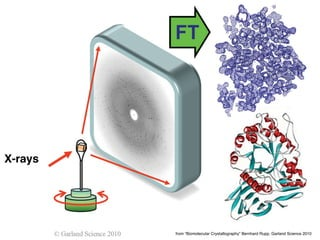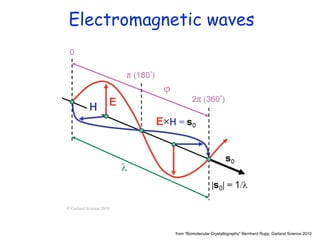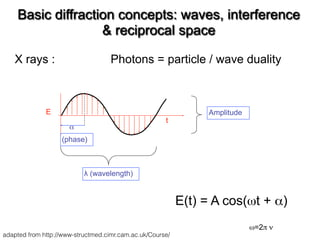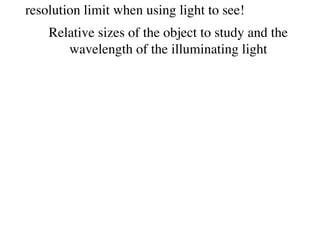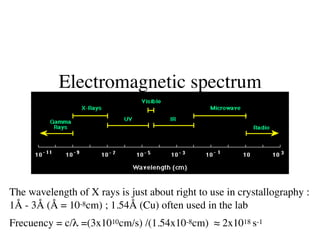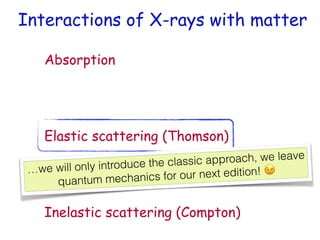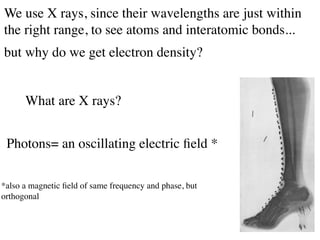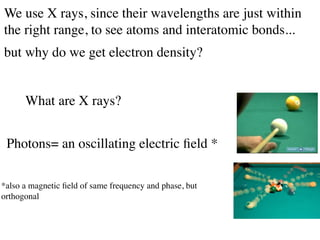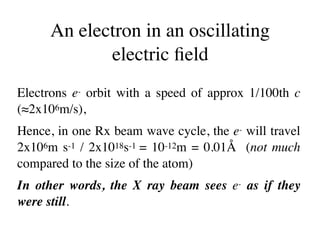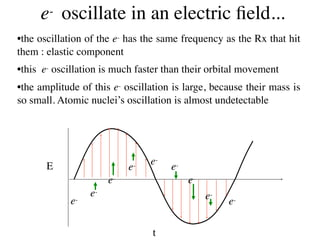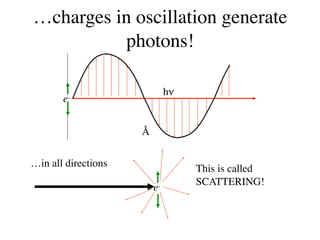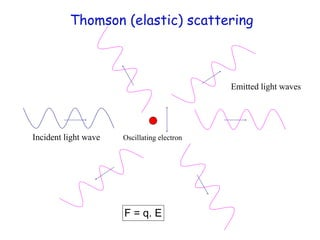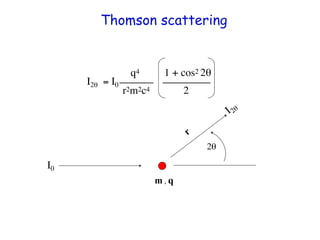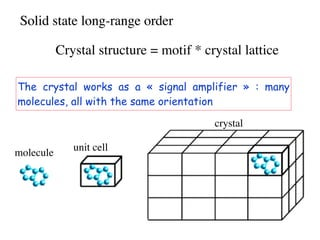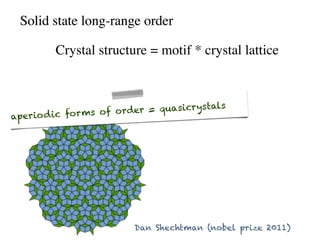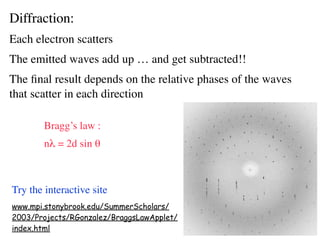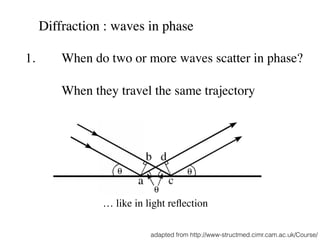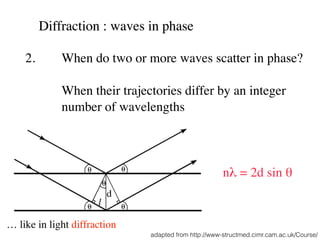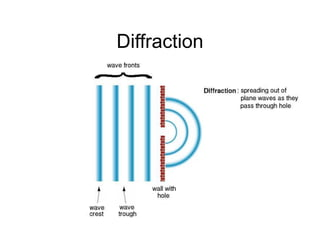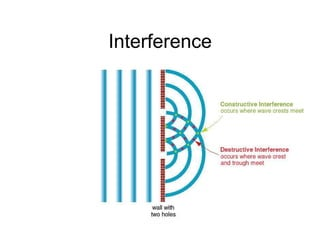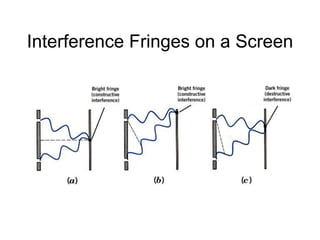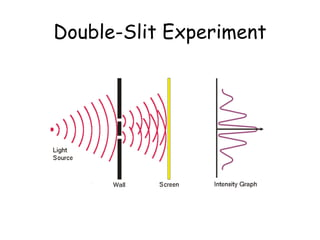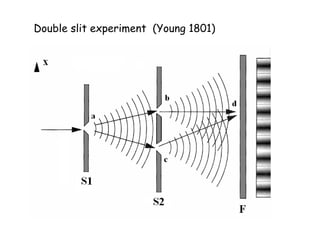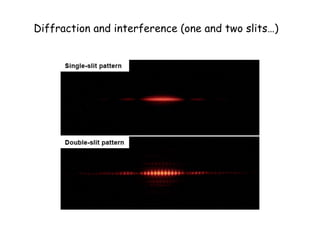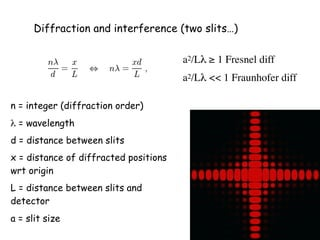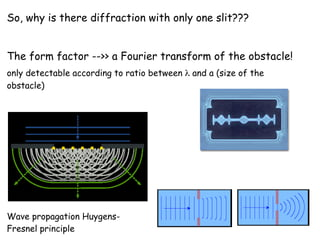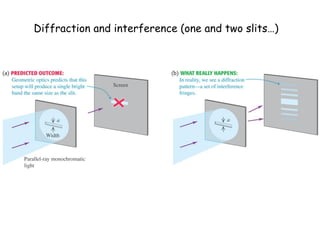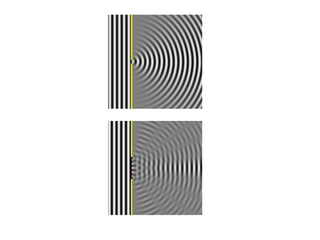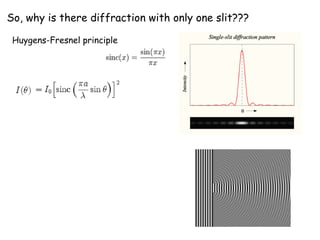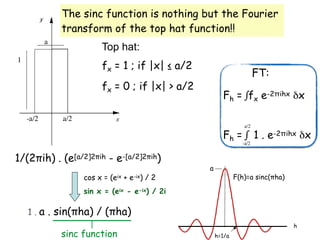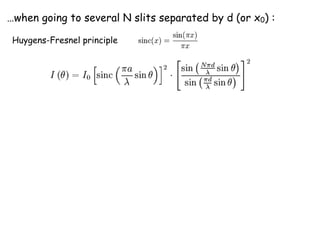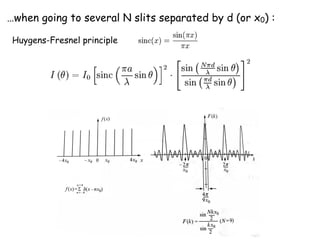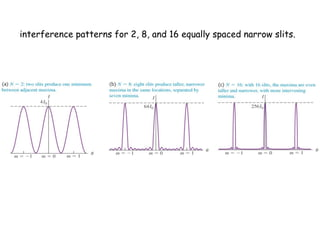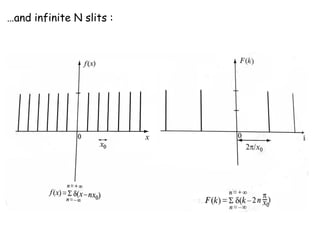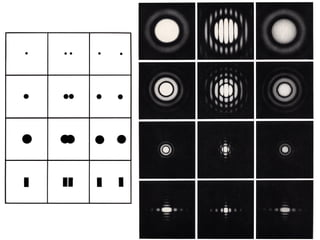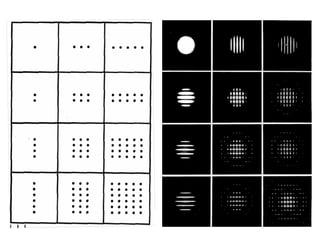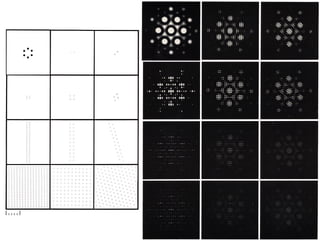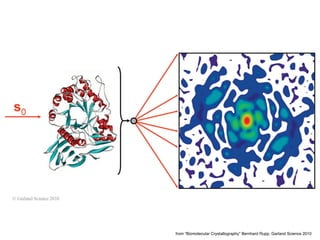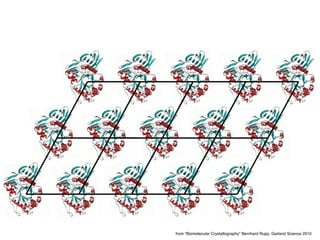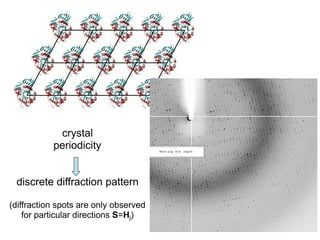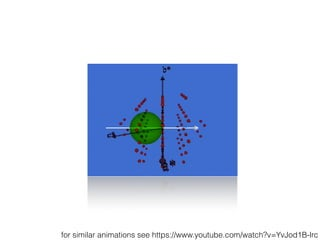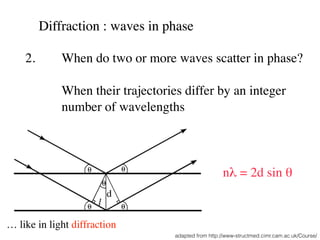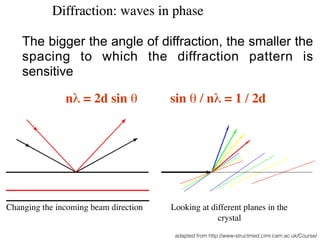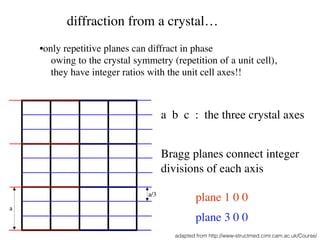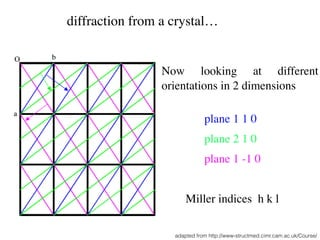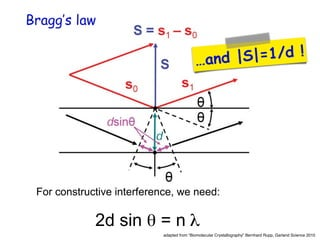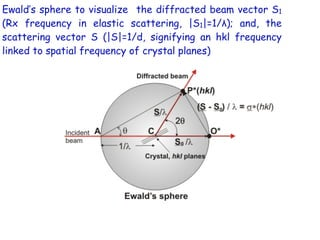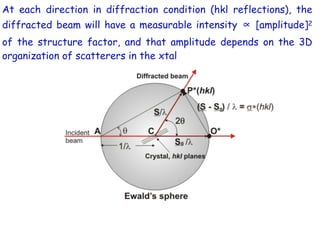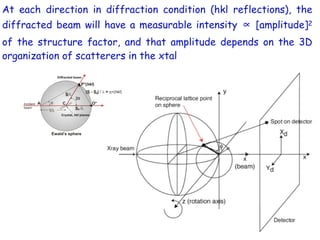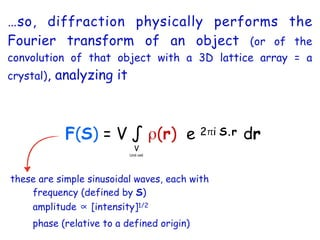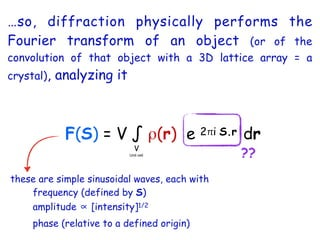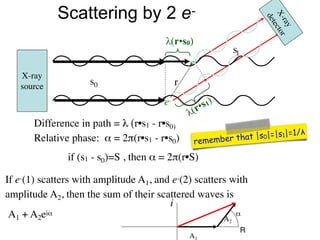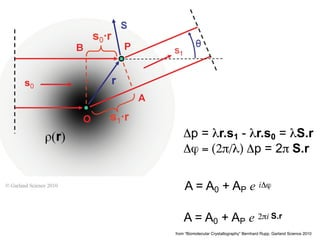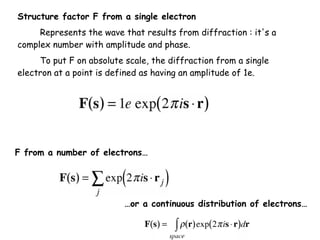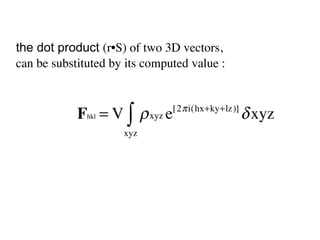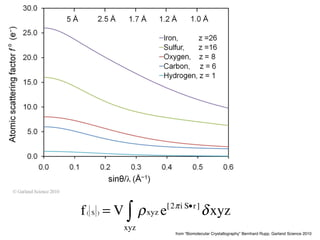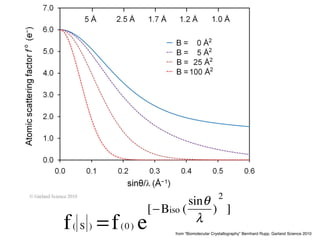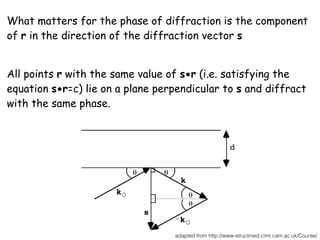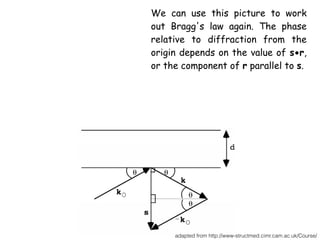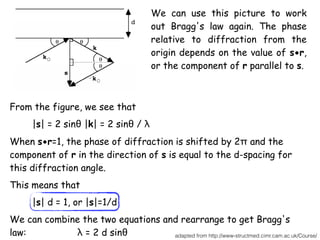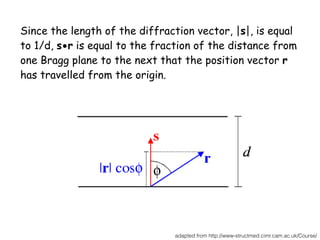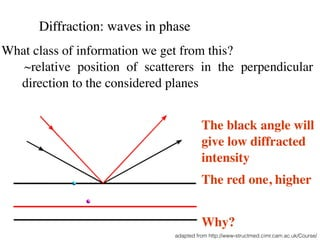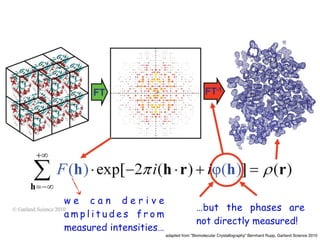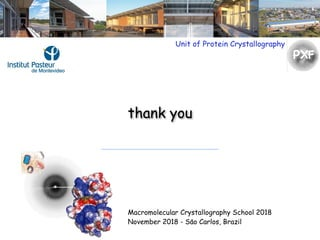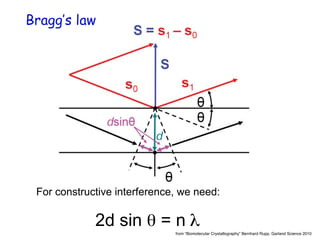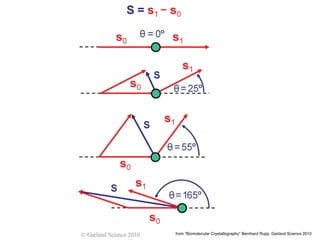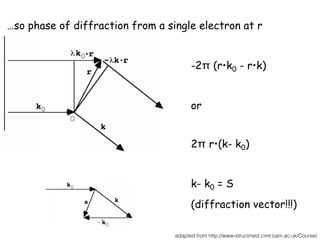1 of 72

### diffraction.pdf

1. Unit of Protein Crystallography Theory of X-ray diffraction PXF Macromolecular Crystallography School 2018 November 2018 - Sāo Carlos, Brazil
2. Diffraction manifests itself as the bending of light trajectory around small obstacles and the “unusual” spreading out of waves past small openings
3. …why would this matter in Biology??? because the pattern produced can be recorded, and gives accurate information about the structure of the material that generated it : molecules and ordered arrays of molecules (such as in crystals), are known to diffract light, uncovering their three-dimensional structure at the atomic level
4. 4 X-rays from “Biomolecular Crystallography” Bernhard Rupp, Garland Science 2010
5. Electromagnetic waves from “Biomolecular Crystallography” Bernhard Rupp, Garland Science 2010
6. Basic diffraction concepts: waves, interference & reciprocal space X rays : E Photons = particle / wave duality Amplitude λ (wavelength) E(t) = A cos(ωt + α) α t ω=2π ν (phase) adapted from http://www-structmed.cimr.cam.ac.uk/Course/
7. E E(x) = A cos(ωx + α) α x ω=2π/λ (phase) Basic diffraction concepts: waves, interference & reciprocal space X rays : Photons = particle / wave duality Amplitude λ (wavelength) adapted from http://www-structmed.cimr.cam.ac.uk/Course/
8. resolution limit when using light to see! Relative sizes of the object to study and the wavelength of the illuminating light
9. Electromagnetic spectrum The wavelength of X rays is just about right to use in crystallography : 1Å - 3Å (Å = 10-8cm) ; 1.54Å (Cu) often used in the lab Frecuency = c/λ =(3x1010cm/s) /(1.54x10-8cm) ≈ 2x1018 s-1
10. Interactions of X-rays with matter Absorption Elastic scattering (Thomson) Inelastic scattering (Compton) …we will only introduce the classic approach, we leave quantum mechanics for our next edition! 😉
11. We use X rays, since their wavelengths are just within the right range, to see atoms and interatomic bonds... but why do we get electron density? What are X rays? Photons= an oscillating electric field * *also a magnetic field of same frequency and phase, but orthogonal
12. We use X rays, since their wavelengths are just within the right range, to see atoms and interatomic bonds... but why do we get electron density? What are X rays? Photons= an oscillating electric field * *also a magnetic field of same frequency and phase, but orthogonal
13. An electron in an oscillating electric field Electrons e- orbit with a speed of approx 1/100th c (≈2x106m/s), Hence, in one Rx beam wave cycle, the e- will travel 2x106m s-1 / 2x1018s-1 = 10-12m = 0.01Å (not much compared to the size of the atom) In other words, the X ray beam sees e- as if they were still.
14. e- oscillate in an electric field... •the oscillation of the e- has the same frequency as the Rx that hit them : elastic component •this e- oscillation is much faster than their orbital movement •the amplitude of this e- oscillation is large, because their mass is so small. Atomic nuclei’s oscillation is almost undetectable E e- e- e- e- e- e- e- e- e- t
15. …charges in oscillation generate photons! e- Å hν …in all directions e- This is called SCATTERING!
16. Thomson (elastic) scattering Oscillating electron Incident light wave Emitted light waves F = q. E
17. q4 1 + cos2 2θ I2θ = I0 r2m2c4 2 Thomson scattering m , q 2θ I2θ I0 r
18. Solid state long-range order molecule unit cell crystal The crystal works as a « signal amplifier » : many molecules, all with the same orientation Crystal structure = motif * crystal lattice
19. Solid state long-range order molecule unit cell crystal The crystal works as a « signal amplifier » : many molecules, all with the same orientation Crystal structure = motif * crystal lattice Dan Shechtman (nobel prize 2011) aperiodic forms of order = quasicrystals
20. Diffraction: Each electron scatters The emitted waves add up … and get subtracted!! The final result depends on the relative phases of the waves that scatter in each direction Try the interactive site www.mpi.stonybrook.edu/SummerScholars/ 2003/Projects/RGonzalez/BraggsLawApplet/ index.html Bragg’s law : nλ = 2d sin θ
21. Diffraction : waves in phase 1. When do two or more waves scatter in phase? When they travel the same trajectory … like in light reflection adapted from http://www-structmed.cimr.cam.ac.uk/Course/
22. … like in light diffraction nλ = 2d sin θ Diffraction : waves in phase 2. When do two or more waves scatter in phase? When their trajectories differ by an integer number of wavelengths adapted from http://www-structmed.cimr.cam.ac.uk/Course/
23. Diffraction
24. Interference
25. Interference Fringes on a Screen
26. Double-Slit Experiment
27. Double slit experiment (Young 1801)
28. Diffraction and interference (one and two slits…)
29. Diffraction and interference (two slits…) n = integer (diffraction order) λ = wavelength d = distance between slits x = distance of diffracted positions wrt origin L = distance between slits and detector a = slit size a2/Lλ ≥ 1 Fresnel diff a2/Lλ << 1 Fraunhofer diff
30. So, why is there diffraction with only one slit??? The form factor -->> a Fourier transform of the obstacle! only detectable according to ratio between λ and a (size of the obstacle) Wave propagation Huygens- Fresnel principle
31. Diffraction and interference (one and two slits…)
32. So, why is there diffraction with only one slit??? Huygens-Fresnel principle
33. Top hat: fx = 1 ; if |x| ≤ a/2 fx = 0 ; if |x| > a/2 The sinc function is nothing but the Fourier transform of the top hat function!! a/2 -a/2 a 1 h h h=1/a h F(h)=a sinc(πha) a 1/(2πih) . (e[a/2]2πih - e-[a/2]2πih) 1 . a . sin(πha) / (πha) cos x = (eix + e-ix) / 2 sin x = (eix - e-ix) / 2i Fh = ∫fx e-2πihx δx Fh = ∫ 1 . e-2πihx δx -a/2 a/2 FT: sinc function
34. …when going to several N slits separated by d (or x0) : Huygens-Fresnel principle
35. …when going to several N slits separated by d (or x0) : Huygens-Fresnel principle
36. interference patterns for 2, 8, and 16 equally spaced narrow slits.
37. …and infinite N slits :
38. from “Biomolecular Crystallography” Bernhard Rupp, Garland Science 2010
39. from “Biomolecular Crystallography” Bernhard Rupp, Garland Science 2010
40. crystal periodicity discrete diffraction pattern (diffraction spots are only observed for particular directions S=Hj)
41. for similar animations see https://www.youtube.com/watch?v=YvJod1B-lrc
42. … like in light diffraction nλ = 2d sin θ Diffraction : waves in phase 2. When do two or more waves scatter in phase? When their trajectories differ by an integer number of wavelengths adapted from http://www-structmed.cimr.cam.ac.uk/Course/
43. Diffraction: waves in phase The bigger the angle of diffraction, the smaller the spacing to which the diffraction pattern is sensitive nλ = 2d sin θ sin θ / nλ = 1 / 2d Changing the incoming beam direction Looking at different planes in the crystal adapted from http://www-structmed.cimr.cam.ac.uk/Course/
44. diffraction from a crystal… a b c : the three crystal axes Bragg planes connect integer divisions of each axis plane 1 0 0 plane 3 0 0 •only repetitive planes can diffract in phase owing to the crystal symmetry (repetition of a unit cell), they have integer ratios with the unit cell axes!! adapted from http://www-structmed.cimr.cam.ac.uk/Course/
45. Now looking at different orientations in 2 dimensions plane 1 1 0 plane 2 1 0 plane 1 -1 0 Miller indices h k l diffraction from a crystal… adapted from http://www-structmed.cimr.cam.ac.uk/Course/
46. For constructive interference, we need: 2d sin θ = n λ Bragg’s law …and |S|=1/d ! adapted from “Biomolecular Crystallography” Bernhard Rupp, Garland Science 2010
47. Ewald’s sphere to visualize the diffracted beam vector S1 (Rx frequency in elastic scattering, |S1|=1/λ); and, the scattering vector S (|S|=1/d, signifying an hkl frequency linked to spatial frequency of crystal planes)
48. At each direction in diffraction condition (hkl reflections), the diffracted beam will have a measurable intensity ∝ [amplitude]2 of the structure factor, and that amplitude depends on the 3D organization of scatterers in the xtal
49. At each direction in diffraction condition (hkl reflections), the diffracted beam will have a measurable intensity ∝ [amplitude]2 of the structure factor, and that amplitude depends on the 3D organization of scatterers in the xtal
50. …so, diffraction physically performs the Fourier transform of an object (or of the convolution of that object with a 3D lattice array = a crystal), analyzing it F(S) = V ∫ ρ(r) e 2πi S.r dr V Unit cell these are simple sinusoidal waves, each with frequency (defined by S) amplitude ∝ [intensity]1/2 phase (relative to a defined origin)
51. …so, diffraction physically performs the Fourier transform of an object (or of the convolution of that object with a 3D lattice array = a crystal), analyzing it F(S) = V ∫ ρ(r) e 2πi S.r dr V Unit cell ?? these are simple sinusoidal waves, each with frequency (defined by S) amplitude ∝ [intensity]1/2 phase (relative to a defined origin)
52. Difference in path = λ (r•s1 - r•s0) Relative phase: α = 2π(r•s1 - r•s0) if (s1 - s0)=S , then α = 2π(r•S) Scattering by 2 e- If e-(1) scatters with amplitude A1, and e-(2) scatters with amplitude A2, then the sum of their scattered waves is A1 + A2eiα A1 A2 α R i remember that |s0|=|s1|=1/λ e- e- r s0 s X-ray source X - r a y d e t e c t o r 1 λ(r•s0) λ(r•s1)
53. Δp = λr.s1 - λr.s0 = λS.r Δϕ = (2π/λ) Δp = 2π S.r A = A0 + AP e iΔϕ A = A0 + AP e 2πi S.r from “Biomolecular Crystallography” Bernhard Rupp, Garland Science 2010
54. Structure factor F from a single electron Represents the wave that results from diffraction : it's a complex number with amplitude and phase. To put F on absolute scale, the diffraction from a single electron at a point is defined as having an amplitude of 1e. F from a number of electrons… …or a continuous distribution of electrons…
55. Fhkl = V ρxyz xyz ∫ e[2πi(hx+ky+lz)] δxyz the dot product (r•S) of two 3D vectors, can be substituted by its computed value :
56. f( S ) = V ρxyz xyz ∫ e[2πi S•r] δxyz from “Biomolecular Crystallography” Bernhard Rupp, Garland Science 2010
57. f( S ) =f(0) e [−Biso ( sinθ λ ) 2 ] from “Biomolecular Crystallography” Bernhard Rupp, Garland Science 2010
58. What matters for the phase of diffraction is the component of r in the direction of the diffraction vector s All points r with the same value of s•r (i.e. satisfying the equation s•r=c) lie on a plane perpendicular to s and diffract with the same phase. adapted from http://www-structmed.cimr.cam.ac.uk/Course/
59. We can use this picture to work out Bragg's law again. The phase relative to diffraction from the origin depends on the value of s•r, or the component of r parallel to s. adapted from http://www-structmed.cimr.cam.ac.uk/Course/
60. From the figure, we see that |s| = 2 sinθ |k| = 2 sinθ / λ When s•r=1, the phase of diffraction is shifted by 2π and the component of r in the direction of s is equal to the d-spacing for this diffraction angle. This means that |s| d = 1, or |s|=1/d We can combine the two equations and rearrange to get Bragg's law: λ = 2 d sinθ We can use this picture to work out Bragg's law again. The phase relative to diffraction from the origin depends on the value of s•r, or the component of r parallel to s. adapted from http://www-structmed.cimr.cam.ac.uk/Course/
61. Since the length of the diffraction vector, |s|, is equal to 1/d, s•r is equal to the fraction of the distance from one Bragg plane to the next that the position vector r has travelled from the origin. adapted from http://www-structmed.cimr.cam.ac.uk/Course/
62. Diffraction: waves in phase What class of information we get from this? ~relative position of scatterers in the perpendicular direction to the considered planes The black angle will give low diffracted intensity The red one, higher Why? adapted from http://www-structmed.cimr.cam.ac.uk/Course/
63. …but the phases are not directly measured! w e c a n d e r i v e a m p l i t u d e s f r o m measured intensities… adapted from “Biomolecular Crystallography” Bernhard Rupp, Garland Science 2010
64. thank you Unit of Protein Crystallography PXF Macromolecular Crystallography School 2018 November 2018 - Sāo Carlos, Brazil
65. For constructive interference, we need: 2d sin θ = n λ Bragg’s law from “Biomolecular Crystallography” Bernhard Rupp, Garland Science 2010
66. from “Biomolecular Crystallography” Bernhard Rupp, Garland Science 2010
67. Again, to look at from a (slightly) different point of view… Incoming : plane wave (vector k) the question is, what is the path in fraction of wavelengths, to point r ? -2π (r•k) / (|k|.λ) -->> -2π (r•k) …if |k|=1/λ adapted from http://www-structmed.cimr.cam.ac.uk/Course/
68. …so phase of diffraction from a single electron at r -2π (r•k0 - r•k) or 2π r•(k- k0) k- k0 = S (diffraction vector!!!) adapted from http://www-structmed.cimr.cam.ac.uk/Course/